Pure and Applied Mathematics Journal
Volume 4, Issue 4, August 2015, Pages: 147-154

Mathematical Methodologies in Physics and Their Applications in Derivation of Velocity and Acceleration Theories

Edward T. H. Wu

Davinci International Academy, Los Angeles, California, USA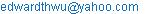Edward T. H. Wu. Mathematical Methodologies in Physics and Their Applications in Derivation of Velocity and Acceleration Theories. Pure and Applied Mathematics Journal. Vol. 4, No. 4, 2015, pp. 147-154. doi: 10.11648/j.pamj.20150404.12

Abstract: The principles to use variables and mathematical methodologies in physics are addressed. A set of refined definitions with designated variables are used to derive the Velocity and Acceleration Theories in Distance Field and Vector Space. Mathematical methodologies such as Linear Algebra and Vector Calculus are used systematically in a step by step derivation process. The proof of the theories can be easily achieved by substitution of the designated variables with a set of parameters that matches the same assumptions and conditions in every step of the derivation process.

Keywords: Variables, Parameters, Velocity, Acceleration, Linear Algebra, Vector Calculus, Mathematical Methodology

Contents

1. Variable and Parameter

A designated variable can be used to represent any individual number (element) of a specific domain in mathematics, or any individual parameter of a specific property of a substance in physics, for the purpose of stating a function, operation and correlation between the domains in mathematics or the properties of a substance in physics. In another word, every definition, principle and theory in mathematics and physics can be represented and correlated by a group of variables as long as each one of them is designated exclusively to a specific domain in mathematics or a specific property of a substance in physics.

2. Mathematical Methodology

The correlations and theories between a group of physical properties with parameters containing numerical quantities and directions, at a specific state and under certain assumptions and conditions, of the same or different substances can be derived by designated variables through the operation and calculation processes of mathematical methodologies such as Linear Algebra (Ref. 1) (Ref. 2) (Ref. 3) (Ref. 4), Vector Calculus (Ref. 5) (Ref. 6) (Ref. 7), etc.

3. Derivation of Theories

A standard procedure to derive a theory in physics involves the following processes:

1.   VARIABLES – Each designated variable is assigned to a property at a specific state of a substance.

2.   IF – Assumptions and conditions are adapted.

3.   FACT–Definitions, principles and theories in mathematics and physics are applied.

4.   DERIVATION–Experience, experiments, logics and mathematical methodologies are used in the derivation processes.

5.   THEORY – Correlations between the properties of the same or different substances are obtained.

Example 1. For a motion in Distance Field, at any initial state (xi, ti) & final state (xf, tf) where xi < xf,

xf – xi =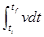[DERIVATION]

1.    VARIABLES

Assign "t" to time, "x" to distance and "v" to velocity. Also assign ti to the initial time, xi to the initial distance and vi to the initial velocity; tf to the final time, xf to the final distance and vf to the final velocity, etc.

2.    IF

The motion in Distance Field is a continuous function F(t) = x, where "x" is distance and "t" is time. At any time "t", there is a corresponding distance "x", together they form a function set (x, t). Similarly at its adjacent time t’, where t’ > t & (t’ – t) = ∆t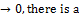corresponding distance x’, also together they form a function set (x’, t’).

3.    FACT

Appling the following definitions:

dt = t’ – t

dx = x’ – x

v = dx/dt

for any two time ti and tf, where ti < tf, we can find (xi, ti) and (xf, tf) and a group of sequential numbers ti, t1, t2, …, tm, tf in the time domain between ti & tf, where (t1 - ti ) = ∆ti→0, (t2 - t1) = ∆t1→0, (t2 - t1) = ∆t2→0, …, (tf - tm ) = ∆tm→0, and also a group of corresponding sequential numbers xi, x1, x2, …, xm, xf in the distance domain between xi & xf, to form a group of function sets (xi, ti), (x1, t1), (x2, t2), …, (xm, tm), (xf, tf), such that:

dti = t1 – ti,     dt1= t2 – t1, …,    dtm = tf – tm

dxi = x1 – xi,    dx1= x2 – x1, …,   dxm= xf – xm

dxi = vidti,    dx1=v1dt1, …,      dxm= vmdtm

4.    DERIVATION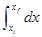= dxi + dx1 +… + dxm = (x1 – xi) + (x2 – x1) +…+ (xf – xm) = xf – xi= vidti + v1dt1 + … + vmdtm

Therefore,

xf – xi =5.    THEORY

The derivation process is completed and the Theory is derived:

For a motion in Distance Field, at any initial state (xi, ti) & final state (xf, tf) where xi < xf,

xf – xi =4. Proof of Theory and Solution

To prove a theory or to verify the solution of a problem, "Substitution Principle" is used with the following procedures:

1. Apply SUBSTITUTION to the designated variables in the derivation or solution process by a set of parameters of the properties at a specific state of the substance that meets the IF conditions and assumptions.

2. Adapt the same FACT and DERIVATION process as that in deriving the theory or solving the problem in every steps of the proving process.

3. PROOF of the theory and solution can be achieved by obtaining the same answer and result at the end of the proving process.

The proof of the Theory and Solution is quite straight forward because the DERIVATION process leads to the same answers and results for every set of the parameters of the properties, at a specific state of the substance, that can satisfy the IF assumptions and conditions.

Example 2. For a motion in Distance Field, If x = 5 t + 2 t2, what is the acceleration?

[SOLVE PROBLEM]

1.    Assign VARIABLE:

"t" is the time from the starting point

∆t > 0 & ∆t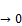t’ = t + ∆t

t" = t’ + ∆t

x = 5 t + 2t2

x’ = 5 t’ + 2(t’) 2

x" = 5 t" + 2 (t") 2

3.    Apply FACT in DERIVATION process:

dt = t’ – t = ∆t

dx = x’ – x = 5 (t’ – t) + 2 ((t’)2 – t2)

= 5∆t + 2 (t2 + 2t∆t + ∆t2 – t2) = 5∆t + 4t ∆t

v = dx/dt = dx/∆t = 5 + 4t

dt’ = t" – t’ = ∆t

dx’ = x" – x’ = 5 (t" – t’) + 2 ((t")2 – (t’)2)

= 5∆t + 2 (t’2 + 2t’∆t + ∆t2 – t’2)= 5∆t + 4t’ ∆t

v’ = dx’/dt’ = dx’/∆t = 5 + 4t’

dv = v’ – v = 4 (t’ – t) = 4 dt

a = dv/dt = 4

4.    Obtain SOLUTION:

The acceleration a = 4.

[PROVE RESULT]

1.    Apply SUBSTITUTION:

Taking any time in the time domain, for example t = 3 sec from the starting point. With a finite ∆t = 0.01 sec, all of the variables at time "t" can thus be substituted as follows:

t = 3

∆t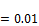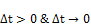t’ = 3 + 0.01

t" = t’ + 0.01= (3 + 0.01) + 0.01

x = 5 + 2 2(2)

x’ = 5 (3 + 0.01) + 2(3 + 0.01) 2

x" = 5 ((3 + 0.01) +0.01) + 2 [(3 + 0.01) +0.01] 2

3.    Apply FACT in DERIVATION process:

dt = t’ – t = (3 + 0.01) – 3 = 0.01

dx = x’ – x = 5 [(3 + 0.01) – 3] + 2 [(3 + 0.01)2 – 32]

= 5 + 2 [2 + 2 + 2 – 32](32)

= 5 + 4(0.01)

v = dx/dt = [5 + 4 ]/ 0.01 = 5 + 4(3)

dt’ = t" – t’ = [(3 + 0.01) + 0.01] - (3 + 0.01) = 0.01

dx’ = x" – x’ = 5 {[(3 + 0.01) + 0.01] – (3 + 0.01)} + 2 {[(3 + 0.01) + 0.01] 2 – (3 + 0.01)2}

= 5 + 2 [(3 + 0.01)2 + 2(3 + 0.01) +2– (3 + 0.01)2](2)

= 5 + 4(3 + 0.01)(0.01)

v’ = dx’/dt’ = [5 + 4(3 + 0.01) ]/ 0.01(0.01)

= 5 + 4(3 + 0.01)

dv = v’ – v = 4[(3 + 0.01) – 3] = 4(0.01)

a = dv/dt = 4/0.01 = 4(4)

4.    Proof of SOLUTION:

Because every step in the proving process is true and also follows exactly the same steps as that in the derivation process, therefore the same results as that of the derivation process can be readily achieved.

All variables can also be replaced by numerical numbers through substitutions and calculations (Table 1). However, it makes no difference to the final results.

Table 1. Substitution of variables with numerical numbers.

 t x t' x' dt=t'-t dx=x'-x v=dx/dt t" x" dt'=t"-t' dx'=x"-x' v'=dx'/dt' dv=v'-v a=dv/dt t1 x1 t1' x1' dt1=t1'-t1 dx1=x1'-x1 v1=dx1/dt1 t1" x1" dt1'=t1''-t1' dx1'=x1"-x1' v1'=dx1'/dt1' dv1=v1'-v1 a1=dv1/dt1 3.000 33.000 3.010 33.170 0.010 0.170 17.020 3.020 33.341 0.010 0.171 17.060 0.040 4.000

5. Motion in Distance Field

The motion of an object in Distance Field (Fig. 1) can be expressed by a function x = F(t). At any time "t", there is a pair of real numbers (x, t) where "t" is the time from the origin, and "x" is the distance along the motion from the origin. Since the starting distance at origin "x0" is 0 and the starting time at origin "t0" is 0, therefore (x0, t0) at origin can be designated as (0, 0).

5.1. Definitions

For a motion in Distance Field x = F(t), at any time "t", there are a corresponding distance "x", and two randomly chosen subsequent times t’ & t", where t < t’ < t" and t’ = t + ∆t (∆t→0) and t" = t’ + ∆t’ (∆t’→0), and their corresponding distances x’ & x". In another word, at any time "t", we can find a position function set (x, t) and two adjacent position function sets (x’, t’) and (x", t"), where t < t’ < t" and t’ = t + ∆t (∆t→0) & t" = t’ + ∆t’ (∆t’→0).

To be more specifically, there is a motion in Distance Field, where x = F(t), such that:

"t" is any time in the time domain of x = F(t)

∆t > 0 and ∆t →0 & ∆t’ > 0 and ∆t’ →0

t → x: find x from t and F(x, t)

t → t’: find t’ from t and ∆t by t’ = t + ∆t

t’→ x’: find x’ from t’ and F(x, t)

t’→ t": find t" from t’ and ∆t’ by t" = t’ + ∆t’

t" → x": find x" from t" and F(x, t)

Giving the above variables t, x, t’, x’, t", and x", we can apply the following definitions:

Differential of Time:

dt = t’ – t            dt’ = t" – t’

Differential of Distance:

dx = x’ – x                  dx’ = x" – x’

Velocity:

v = dx/dt           v’ = dx’/dt’

dx = vdt             dx’ = v’dt

Differential of Velocity:

dv = v’ – v

Acceleration:

a = dv/dt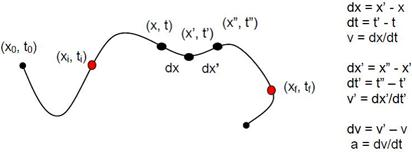Fig. 1. Motion in Distance Field.

5.2. Velocity

5.2.1. Theory 1

For a motion in the Distance Field, at any two time ti and tf (ti < tf) in the time domain, there are two corresponding distances xi and xf such that:

xf – xi ==(1)

Also, for a motion in the Distance Field with origin (0, 0), at any time t in the time domain, there is a corresponding distance x such that:

x =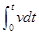(2)

[PROOF]

For a motion in the Distance Field x = F(t), at any time "t", there are (x, t) and (x’, t’), where t’ > t & (t’ – t) = ∆t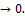Also by applying the following definitions:

dt = t’ – t

dx = x’ – x

dx = vdt

for a motion in Distance Field, at any two times ti and tf that ti < tf, we can find (xi, ti) and (xf, tf) and a group of continuous sequential numbers ti, t1, t2, …, tm, tf in the time domain between ti & tf, where (t1 – ti ) = ∆ti→0, (t2 – t1) = ∆t1→0, (t2 – t1) = ∆t2→0, …, (tf –tm ) = ∆tm→0, and also a group of corresponding continuous sequential numbers xi, x1, x2, …, xm, xf in the distance domain between xi & xf, to form position function sets (xi, ti), (x1, t1), (x2, t2), …, (xm, tm), (xf, tf), such that:

dti = t1– ti,    dt1= t2 – t1, …,   dtm = tf – tm

dxi = x1 – xi,   dx1= x2 – x1, …,   dxm= xf – xm

dxi = vidti,   dx1= v1dt1, …,   dxm= vmdtm= dxi + dx1 +… + dxm = (x1 – xi) + (x2 – x1) +… + (xf – xm) = xf – xi= vidti + v1dt1 + … + vmdtm

Therefore,

For any (xi, ti) & (xf, tf)

xf – xi ==For any (x, t) with origin (0, 0), where xi = 0 & ti = 0 and xf = x & tf = t

x =5.2.2. Theory 2

For a motion in Distance Field, at any two time ti and tf, (ti < tf) in the time domain, there are two corresponding distances xi and xf such that:

For a constant velocity v,

xf – xi = v(tf – ti)               (3)

Also, for a motion in Distance Field with origin (0, 0), for any time t in the time domain, there is a corresponding distance x such that:

For a constant velocity v,

x = vt                   (4)

[PROOF]

Because v is a constant, v = vi = v1 = v2 =… = vm= vidti + v1dt1 + … + vmdtm = vdti + vdt1 + vdt2 +… +vdm = v (dti + dt1 + dt2 + …+ dtm) = v (tf – ti)= dxi + dx1 +… +dxm = xf - xi=Therefore, for any (xi, ti) & (xf, tf), and constant v,

xf – xi = v(tf – ti)

For any time t with origin (0, 0), and constant v, where xi = 0 & ti = 0 and xf = x & tf = t

x= vt

5.3. Acceleration

5.3.1. Theory 1

For a motion in Distance Field, at any two times ti and tf, (ti < tf) in the time domain, there are two corresponding velocities vi and vf such that: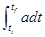= vf – vi           (5)

Also, for a motion in Distance Field with origin (0, 0), at any time t in the time domain, there is a corresponding velocity v such that: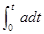= v – v0            (6)

[PROOF]

For a motion in Distance Field, at any time t, there are (x, t), (x’, t’) and (x", t") where t’ > t & (t’ – t) = ∆tAlso by applying the following definitions:

dt = t’ – t, dt’ = t" – t’

dx = x’ – x , dx’ = x" – x’

v = dx/dt , v’ = dx’/dt’

dv = v’ – v

for a motion in Distance Field, at any two times ti and tf, that ti < tf, we can find (xi, ti) and (xf, tf) and a group of continuous sequential numbers ti, t1, t2, …, tm, tf in the time domain between ti & tf, where (t1 – ti ) = ∆ti→0, (t2 – t1) = ∆t1→0, (t2 – t1) = ∆t2→0, …, (tf– tm ) = ∆tm→0, and also a group of corresponding continuous sequential numbers xi, x1, x2, …,xm, xf in the distance domain between xi & xf, to form position function sets (xi, ti), (x1, t1), (x2, t2), …, (xm, tm), (xf, tf), such that:

dti = t1 – ti, dt1= t2 – t1, …, dtm = tf – tm

dxi = x1 – xi, dx1= x2 – x1, …, dxm= xf – xm

vi = dxi/dti, v1= dx1/dt1, …, vm = dxm/dtm

dvi = v1 – vi, dv1 = v2 – v1, …, dvm = vf – vm

ai = dvi/dti, a1 = dv1/dt1, …, am = dvm/dtm

dvi = aidti, dv1 = a1dt1, …, dvm = amdtm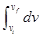= dvi + dv1 +… + dvm = (v1 – vi) + (v2 – v1) +…

+ (vf – vm) = vf – vi= aidti + a1dt1 + … + amdtm

Therefore,

For any (vi, ti) & (vf, tf)= vf – vi

For any time t with origin (0, 0), where xi = 0, ti = 0, vi = v0 and xf = x, tf = t, vf = v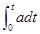= v – v0

5.3.2. Theory 2

For a motion in Distance Field, at any two times ti and tf (ti < tf) in the time domain, there are two corresponding velocities vi and vf such that:

For a constant acceleration a,

vf – vi = a(tf – ti)                (7)

Also, for a motion in the Distance Field with origin (0, 0), at any time t in the time domain, there is a corresponding velocity v such that:

For a constant acceleration a,

v = v0 + at                  (8)

[PROOF]

Because,

vf – vi =a = ai = a1 = a2 = … = am

vf – vi == aidti + a1dt1 + … + amdtm

Therefore, for any (vi, ti) & (vf, tf), and a constant acceleration a,

vf – vi = a(tf – ti)

Also, for any time t with origin (0, 0), and a constant acceleration a, where xi = 0, ti = 0, vi = v0 and xf = x, tf = t, vf = v, then

v = v0 + at

5.3.3. Theory 3

For a motion in Distance Field with origin (0, 0), at any time t in the time domain, there is a corresponding distance x such that:

For a constant acceleration a,

x = v0t + ½ at2               (9)

[PROOF]

Because for a motion in Distance Field with origin (0, 0), at any time t in the time domain, for a constant acceleration a,

v = v0 + at

x =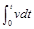x ==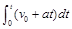=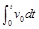+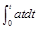= v0t +[Theory]

d(xn) = nxn-1dx

d(xn) = (x’)n – xn = (x + dx)n – xn = (xn + nxn-1dx + ?dx2 + …) – xn = nxn-1dx

Therefore,=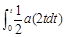=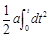=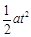x = v0t + ½ at2

5.3.4. Theory 4

For a motion in Distance Field with origin (0, 0), at any time t in the time domain, there is corresponding distance x and velocity v such that:

For a constant acceleration a,

v2 = v02+ 2 ax             (10)

[PROOF]

Because,

v = v0 + at

t = (v – v0)/a

x = v0t + ½ at2

x = v0[(v – v0)/a] + ½ a (v – v0)2/a2= vv0/a – v02/a + ½ (v2– 2v v0+ v02)/a = ½ (v2 – v02)/a

Therefore,

v2 = v02 + 2ax

5.3.5. Theory 5

For a motion in Distance Field with origin (0, 0), at any time t in the time domain, there is a corresponding distance x and velocity v such that:

For a constant acceleration a,

x = ½ (v + v0) t               (11)

[PROOF]

Because,

v = v0 + at

x = v0t + ½ at2

x = ½ v0t + (½ v0t + ½ at2) = ½ v0t + ½ vt

Therefore,

x = ½ (v + v0) t

6. Motion in Vector Space

The motion of an object in Vector Space (Fig. 2) (Ref. 8) (Ref. 9) (Ref. 10) can be expressed by a continuous function X = F(t). At any time "t", there is a pair of position vector and real number (X, t) where "t" is the time from the origin and "X" is the position vector from the origin. Since the starting position vector at origin "X0" is "0" and the starting time at origin "t0" is 0, therefore (X0, t0) at origin can be designated as (0, 0).

6.1. Definitions

For a motion in Vector Space, at any time "t" , there are a corresponding position vector "X", and two randomly chosen subsequent times t’ & t" where t < t’ < t" and t’ = t + ∆t (∆t→0) and t" = t’ + ∆t’ (∆t’→0), and their corresponding position vectors X’ & X". In another word, at any time "t", we can find a position function set (X, t) and two adjacent position function sets (X’, t’) and (X", t"), where t < t’ < t" and ∆t & ∆t’ →0.

To be more specifically, there is a motion in Vector Space, where X = F(t), such that:

"t" is any time in the time domain of X = F(t)

∆t > 0 and ∆t →0 & ∆t’ > 0 and ∆t’ →0

t → X: find X from t and F(X, t)

t → t’: find t’ from t and ∆t by t’ = t + ∆t

t’→ X’: find X’ from t’ and F(X, t)

t’→ t": find t" from t’ and ∆t’ by t" = t’ + ∆t’

t" → X": find X" from t" and F(X, t)

With the above variables t, X, t’, X’, t", and X", we can apply the following definitions:Differential of Time:

dt = t’ – t            dt’ = t" – t’

Differential of Position Vector:

dX = X’ – X                dX’ = X" – X’

Velocity Vector:

V = dX/dt          V’ = dX’/dt’

dX = Vdt            dX’ = V’dt’

Differential of Velocity Vector:

dV = V’ – V

Acceleration Vector:

A = dV/dtFig. 2. Motion in Vector Space.

Replace the real umber variables x, dx, v, dv, a in the motion of Distance Field by corresponding vector variables X, dX, V, dV, A in the motion of Vector Space as follows:

x → X

dx → dX

v → V

dv → dV

a → A

Then, all the derivation processes and theories in the motion of Vector Space will be exactly identical as that in the motion of Distance Field.

6.2. Velocity

6.2.1. Theory 1

For a motion in Vector Space, at any two time ti and tf (ti < tf), there are two corresponding position vectors Xi and Xf such that:

Xf – Xi =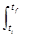Vdt             (12)

Also, for a motion in Vector Space with origin (0, 0), at any time t there is a corresponding position vector X such that:

X =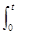Vdt                (13)

6.2.2. Theory 2

For a motion in Vector Space, for any two time ti and tf (ti < tf) in the time domain, there are two corresponding position vectors Xi and Xf such that:

For a constant velocity vector V,

Xf – Xi = V (tf – ti)            (14)

Also, for a motion in Vector Space with origin (0, 0), at any time t in the time domain there is a corresponding position vector X such that:

For a constant velocity vector V,

X = V t                 (15)

6.3. Acceleration

6.3.1. Theory 1

For a motion in Vector Space, at any two time ti and tf (ti < tf) in the time domain, there are two corresponding velocity vectors Vi and Vf such that:Adt = Vf – Vi                  (16)

For a motion in Vector Space with origin (0, 0), at any time t in the time domain, there is a corresponding velocity vector V such that: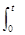Adt = V – V0                (17)

6.3.2. Theory 2

For a motion in Vector Space, at any two time ti and tf (ti < tf) in the time domain, there are two corresponding velocity vectors Vi and Vf such that:

For a constant acceleration vector A,

Vf – Vi = A (tf – ti)               (18)

For a motion in Vector Space with origin (0, 0), at any time t in the time domain, there is a corresponding velocity vector V such that:

For a constant acceleration vector A,

V = V0 + A t                  (19)

6.3.3. Theory 3

For a motion in Vector Space with origin (0, 0), at any time t in the time domain, there is a corresponding position vector X such that:

For a constant acceleration vector A,

X = V0 t + ½ A t2                (20)

6.3.4. Theory 4

For a motion in Vector Space with origin (0, 0), at any time t in the time domain, there is a position vector X and velocity vector V such that:

For a constant acceleration vector A,

V2 = V02+ 2 AX                 (21)

6.3.5. Theory 5

For a motion in Vector Space with origin (0, 0), at any time t in the time domain, there is a position vector X and velocity vector V such that:

For a constant acceleration vector A,

X = ½ (V + V0) t               (22)

References

1. Strang, Gilbert (July 19, 2005), Linear Algebra and Its Applications (4th ed.), Brooks Cole, ISBN 978-0-03-010567-8.
2. Weisstein, Eric. "Linear Algebra". From MathWorld--A Wolfram Web Resource. Wolfram. Retrieved 16 April 2012.
3. Vitulli, Marie."A Brief History of Linear Algebra and Matrix Theory".Department of Mathematics. University of Oregon. Archived fromthe originalon 2012-09-10. Retrieved 2014-07-08.
4. http://en.wikipedia.org/wiki/Linear_algebra.
5. Galbis, Antonio & Maestre, Manuel (2012).Vector Analysis Versus Vector Calculus. Springer. p. 12.ISBN978-1-4614-2199-3.
6. J.E. Marsden (1976). Vector Calculus. W. H. Freeman & Company. ISBN 0-7167-0462-5.
7. Michael J. Crowe (1967). A History of Vector Analysis : The Evolution of the Idea of a Vectorial System. Dover Publications; Reprint edition. ISBN 0-486-67910-1.
8. Bourbaki, Nicolas (1987), Topological vector spaces, Elements of mathematics, Berlin, New York: Springer-Verlag, ISBN 978-3-540-13627-9.
9. Bourbaki, Nicolas (2004), Integration I, Berlin, New York: Springer-Verlag, ISBN 978-3-540-41129-1.
10. Braun, Martin (1993), Differential equations and their applications: an introduction to applied mathematics, Berlin, New York: Springer-Verlag, ISBN 978-0-387-97894-9.

 Contents 1. 2. 3. 4. 5. 5.1. 5.2. 5.3. 6. 6.1. 6.2. 6.3.
Article ToolsAbstractPDF(404K)